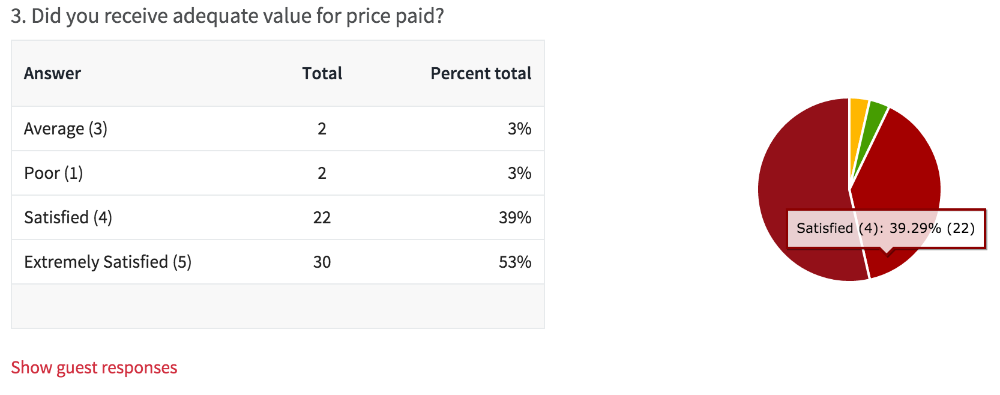# How survey response scores are calculated

Surveys can generate a lot of response data and associated aggregate statistics. Here you will find detailed explanation of how these totals and percentages are calculated.

### Calculation of response percentages for Rating type question

Here is an explanation of how the response percentages are calculated; if it still needs clarifying please let us know.

Each question that requires a rating type response has multiple possible answers, with a score associated with each answer. For example, consider the following rating question with 4 answers, scored from 3 to 0:

Overall, I was satisfied with my stay at Hotel Perfect:

• Far exceeded expectations (worth 3 points)
• Exceeded expectations (worth 2 points)
• Met expectations (worth 1 point)
• Did not meet expectations (worth 0 points)

The percentage number next to each rating in the survey report represents the percentage of total respondents that selected this answer. For example, if 100 people answered the question and 10 of them selected "exceeded expectations" then the stats would show 10%.

What does the percentage number next to the pie chart mean?

1. The percentage number shows the total satisfaction score for that question.

2. This is calculated by totalling the number of points scored (e.g. 3 points for every "far exceeded expectations", 2 for every "exceeded expectations" etc) and expressing it as a percentage of the total number of points possible if everyone answered "far exceeded expectations".

3. For example if 10 people answered the question, 3 selected "far exceeded" (3 x 3 = 9 points), 4 selected "exceeded" (4 x 2 = 8 points), 2 selected "met" (2 x 1 = 2 points) and 1 selected "did not meet" (0 points), your points total for that question is 9 + 8 + 2 + 0 = 19 out of a possible 30. The percentage (total satisfaction score) is then calculated as 19/30 x 100 = 63%.

N/A responses are totally excluded from the total score. That way they don't distort the score given by responses actually answering the question.